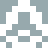### Real Vim ninjas count every keystroke - do you?

###### Pick a challenge, fire up Vim, and show us what you got.

```Your VimGolf key: please sign in

\$ gem install vimgolf
\$ vimgolf setup
\$ vimgolf put 9v0064a68333000000000254
```

### Find fix point of cos

Write a function to find the fix point of cos. It is not a good code; It is just to use vim. Inspired by the excellent https://mvanier.livejournal.com/2897.html about the Y combinator.

##### Start file
```from math import cos

def find_cos_fix_point(j):
a = 12.216
for i in range(j):
a = cos(a)

print(a)

find_cos_fix_point(1)
```
##### End file
```from math import cos

def find_cos_fix_point(j):
a = 12.216
for i in range(j):
a = cos(a)

print(a)

find_cos_fix_point(1)
find_cos_fix_point(10)
find_cos_fix_point(100)
find_cos_fix_point(1000)
find_cos_fix_point(10000)
find_cos_fix_point(100000)
find_cos_fix_point(1000000)
find_cos_fix_point(10000000)
find_cos_fix_point(100000000)
```

#### View Diff

```12a13,20
> find_cos_fix_point(10)
> find_cos_fix_point(100)
> find_cos_fix_point(1000)
> find_cos_fix_point(10000)
> find_cos_fix_point(100000)
> find_cos_fix_point(1000000)
> find_cos_fix_point(10000000)
> find_cos_fix_point(100000000)
```

### Solutions by @xx19971016:

Unlock 4 remaining solutions by signing in and submitting your own entry

## 185 active golfers, 661 entries

##### Solutions by @xx19971016:14
###### #22 - 李建宏 / @xx19971016

08/28/2023 at 08:22AM15
###### #>92 - 李建宏 / @xx19971016

08/28/2023 at 08:16AM16
###### #>117 - 李建宏 / @xx19971016

08/28/2023 at 08:14AM60
###### #>166 - 李建宏 / @xx19971016

08/28/2023 at 08:08AM